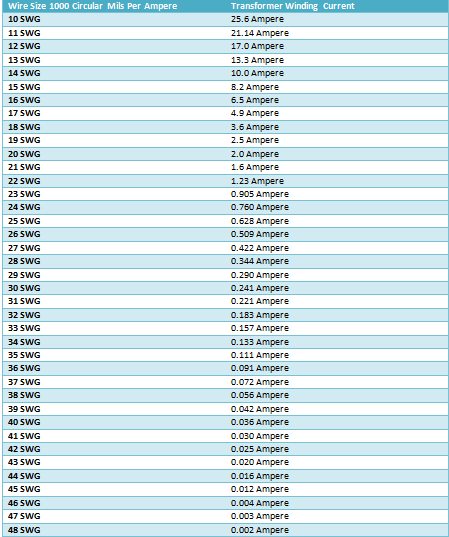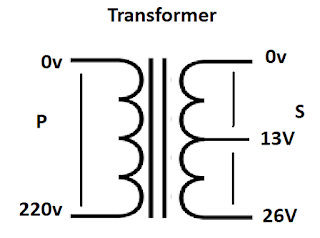# How to Choose Suitable Wire Size for Transformer Winding

Today I am sharing with you the simple method of choosing the suitable wire size for transformer for winding. Or how to chose the wire size for transformer winding. To chose the suitable wire size for transformer primary winding and secondary winding is first and mine point of designing a transformer. Today I will share with you simple table which help you to select the wire size for a transformer.

As you know that we already talk about the turn per volt formula. however in this post we will also use in example to make it easy to understand. And if you would like to learn it complete then watch the below video tutorial first in Urdu/Hindi language.

## How to chose the wire size for transformer winding?

Before choosing a transformer we need to think about the some main points…
For example

### The working time of transformer

Before to chose the wire size for transformer, you need to find out how much time the transformer will work. If your transformer work for 12 hours then you need to chose the wire size according the 12 hours time and if the transformer working time is 4 to 6 hours then you need to select the wire size according the 6 hours time….

### The installation place of transformer

You must be think about the transformer installation. mostly we fix he transformer in box. So if the transformer install in box. Then air flow in box. Also don't install transformer near other machine which produce the heat….

Here I am sharing with a transformer winding current table with transformer wire size gouges according the 1000 circular mils per ampere with transformer winding current. This is suitable for a transformer which is used for 4 to 6 hours. And if you want to use the transformer more than 6 hours then use a number high gauge.### How to select the suitable wire size for transformer winding

Here I clear everything using an example.

### Transformer Wire Size Selection

For example: Bobbin Size 1.5” x 2” L x W = 3
Primary voltage is : 220V
Secondary Voltage is : 13V + 13V
Secondary Current is : 7A
Primary current : ?
First Find out the Primary current
Formula : SI x SE / PE …. So 8.2 x 26 / 220 = 0.969A### Primary Turns & Secondary Turns Per Volt = ?

Bobbin Size 1.5” x 2” = 3 So 7.5/3 = 2.5 turn per volt
But Due to Loses we get 8/3= 2.66 turn per volt
Primary Turns is 2.66 x 220 = 586
Secondary Turns 2.66 x 13 = 35 + 35
According To Table 1000 Circular Mils
Suitable wire size for Secondary is 15 SWG
And For Primary 22 SWG

#### Watch Video Tutorial

Now i hope you will complete understood the chose the suitable wire size for transformer winding. Now if you have question then you use the below comment section..
NEXT ARTICLE Next Post
PREVIOUS ARTICLE Previous Post
NEXT ARTICLE Next Post
PREVIOUS ARTICLE Previous Post